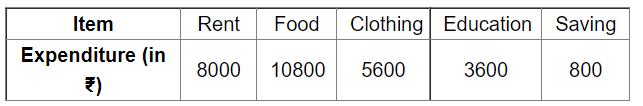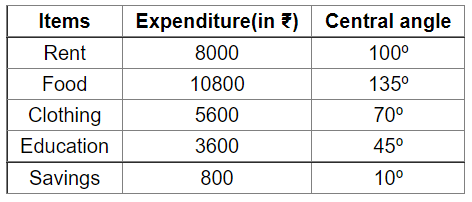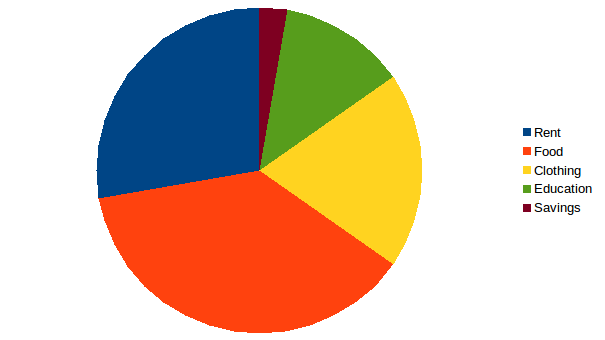# The monthly income of family is ₹ 28800. The monthly expenditure of the family on various items is given below.

Question:

The monthly income of family is ₹ 28800. The monthly expenditure of the family on various items is given below.Represent the above data by a pie chart.

Solution:

Monthly income of the family = ₹28,800

Central angle for each item $=\left(\frac{\text { Expenditure per month on various items }}{\text { Monthly income }} \times 360\right)^{\circ}$

Calculation of central anglesConstruction of pie chart
Steps of construction

1. Draw a circle of any convenient radius.

2. Draw a horizontal radius of this circle.

3. Draw sectors whose central angles are 100º, 135º, 70º, 45º and 10º.

4. Shade the sectors so obtained differently and label each one of them.

Thus, the pie chart is obtained as follows.